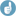## CV from CI [Power / Sample Size]

Hi Louis,

❝ […] how to get the CV(intra) when little or no (or not reliable) prior info available. I went over the "Biostatistics Sample Size Estimation for BE Studies" presentation […] and at one point in one of the "Hints" slide it says that "All you need is the 90% geometric confidence interval and the sample size."

I guess you are referring to this presentation (slide 24), right?

❝ Am I understanding correctly that the CLlow and CLhigh would be the 90% confidence limits for a prior CV intra…

Essentially yes.

<nitpick>

The 100(1–2α) CI is calculated from

1. the geometric mean ratio (GMR),
2. the number of subjects / sequence,
3. the t-value (for given α and degrees of freedom specific for the design), and
4. the mean square error (which is an estimate of the variance).

It’s not rocket science to perform the calculation “backwards” (get the unknown #4 from the known #1–#3 and the CI) and then $$CV_{intra} = \sqrt{e^{MSE} - 1}$$. If the GMR is not known, use $$\sqrt{CL_{lower} \times CL_{upper}}$$.

</nitpick>

❝ I'm asking that because of the "...geometric confidence interval...". Why are we talking here about that?

I wanted to make clear that we need for the calculations outlined in slides 25–28 the confidence interval derived from log-transformed data (which is required for most PK metrics like AUC, Cmax,…).
Note that the calculation in the presentation is outlined for 2×2 crossover designs. It can be a little bit tricky to perform the calculations “manually” if you are unsure how the degrees of freedom in other designs are defined. I recommend the package PowerTOST for R (open source & free of costs).Examples:
• 2×2 crossover, subjects in the two sequence (n1, n2) 11 and 10 (planned for 22 but one dropout), α 0.05 (90% CI), lower CL 0.91, upper CL 1.15: Calculate the CVintra and then the CI in order whether the input was correct (should give the original CI).
library(PowerTOST) alpha  <- 0.05 lower  <- 0.91 upper  <- 1.15 n      <- c(11, 10) design <- "2x2" CV     <- CVfromCI(alpha=alpha, lower=lower, upper=upper,                    n=n, design=design) CI     <- CI.BE(alpha=alpha, pe=sqrt(lower*upper), CV=CV,                 n=n, design=design) cat("\n CV =", sprintf("%.2f%%", 100*CV),     "\n", sprintf("%g%%", 100*(1-2*alpha)), "CI =",     paste(CI, collapse = " to "), "\n")
Should give
 CV = 22.17%  90% CI = 0.91 to 1.15
• As above but the study was a parallel design in 2×20 subjects. Use n <- c(20, 20) and design <- "parallel" to get
 CV = 22.22%  90% CI = 0.91 to 1.15
Note that this is the total (or pooled) CV and >2 groups are not supported by the function.
• If the study was a replicate design for reference-scaling evaluated for Average Bioequivalenc with Expanding Limits (ABEL: EMA, Health Canada, Russia, and many others): You need only the upper expanded limit (neither the CI, the design, or the sample size).
U  <- 1.38 CV <- CVwRfromU(U=U) cat("\n CVwR =", sprintf("%.2f%%", 100*CV), "\n")
Should give
 CVwR = 44.35%
Note that CVwR is the within-subject CV of the reference product.

Dif-tor heh smusma 🖖🏼 Довге життя Україна!Helmut SchützThe quality of responses received is directly proportional to the quality of the question asked. 🚮
Science QuotesIng. Helmut Schütz